# HSSlive: Plus One & Plus Two Notes & Solutions for Kerala State Board

## BSEB Class 9 Maths Chapter 4 Linear Equations in Two Variables Ex 4.3 Textbook Solutions PDF: Download Bihar Board STD 9th Maths Chapter 4 Linear Equations in Two Variables Ex 4.3 Book AnswersBSEB Class 9 Maths Chapter 4 Linear Equations in Two Variables Ex 4.3 Textbook Solutions PDF: Download Bihar Board STD 9th Maths Chapter 4 Linear Equations in Two Variables Ex 4.3 Book Answers

BSEB Class 9th Maths Chapter 4 Linear Equations in Two Variables Ex 4.3 Textbooks Solutions and answers for students are now available in pdf format. Bihar Board Class 9th Maths Chapter 4 Linear Equations in Two Variables Ex 4.3 Book answers and solutions are one of the most important study materials for any student. The Bihar Board Class 9th Maths Chapter 4 Linear Equations in Two Variables Ex 4.3 books are published by the Bihar Board Publishers. These Bihar Board Class 9th Maths Chapter 4 Linear Equations in Two Variables Ex 4.3 textbooks are prepared by a group of expert faculty members. Students can download these BSEB STD 9th Maths Chapter 4 Linear Equations in Two Variables Ex 4.3 book solutions pdf online from this page.

## Bihar Board Class 9th Maths Chapter 4 Linear Equations in Two Variables Ex 4.3 Books Solutions

 Board BSEB Materials Textbook Solutions/Guide Format DOC/PDF Class 9th Subject Maths Chapter 4 Linear Equations in Two Variables Ex 4.3 Chapters All Provider Hsslive

## How to download Bihar Board Class 9th Maths Chapter 4 Linear Equations in Two Variables Ex 4.3 Textbook Solutions Answers PDF Online?

2. Click on the Bihar Board Class 9th Maths Chapter 4 Linear Equations in Two Variables Ex 4.3 Answers.
3. Look for your Bihar Board STD 9th Maths Chapter 4 Linear Equations in Two Variables Ex 4.3 Textbooks PDF.
4. Now download or read the Bihar Board Class 9th Maths Chapter 4 Linear Equations in Two Variables Ex 4.3 Textbook Solutions for PDF Free.

## BSEB Class 9th Maths Chapter 4 Linear Equations in Two Variables Ex 4.3 Textbooks Solutions with Answer PDF Download

Find below the list of all BSEB Class 9th Maths Chapter 4 Linear Equations in Two Variables Ex 4.3 Textbook Solutions for PDF’s for you to download and prepare for the upcoming exams:

## BSEB Bihar Board Class 9th Maths Solutions Chapter 4 Linear Equations in Two Variables Ex 4.3

Question 1.
Draw the graph of each of the following linear equations in two variables :
(i) x + y = 4
(ii) x – y = 2
(iii) y = 3x
(iv) 3 = 2x + y
Solution:
(i) We have, x + y = 4 ⇒ y = 4 – x
When x = 0, we have : y = 4 – 0 = 4
When x = 2, we have : y = 4 – 2 = 2
When x = 4, we have : y = 4 – 4 = 0
Thus, we have the following table :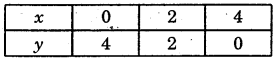Plotting the points (0, 4), (2, 2) and (4, 0) on the graph paper and drawing a line joining them, we obtain the graph of the line represented by the given equation as shown.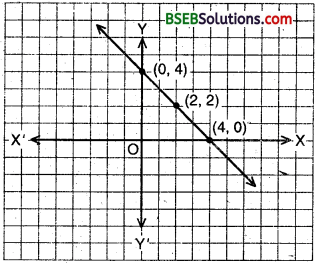(ii) We have, x – y = 2 ⇒ y = x – 2
When x = 0, we have y = 0 – 2 = – 2
When x = 2, we have y = 2 – 2 = 0
When x = 4, we have y = 4 – 2 = 2
Thus, we have the following table :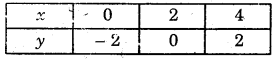Plotting the points (0, – 2), (2, 0) and (4, 2) on the graph paper and drawing a line joining them, we obtain the graph of the line represented by the given equation as shown.(iii) We have, y = 3x ,
When x = 0, we have : y = 3(0) = 0
When x = 1, we have : y = 3(1) = 3
When x = – 1, we have : y = 3(- 1) = – 3
Thus, we have the following table :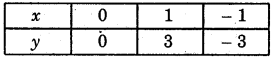Plotting the points (0, 0), (1, 3) and (- 1, – 3) on the graph paper and drawing a line joining them, we obtain the graph of the line represented by the given equation as shown.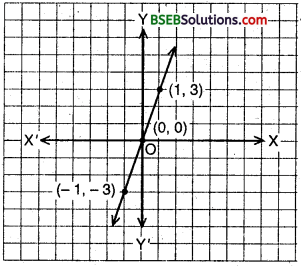(iv) We have, 3 = 2x + y ⇒ y = 3 – 2x
When x = 0, we have :
y = 3 – 2(0) = 3 – 0 = 3
When x = 3, we have :
y = 3 – 2(3) = 3 – 6 = – 3
When x = – 1, we have :
y = 3 – 2(- 1) = 3 + 2 = 5
Thus, we have the following table :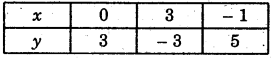Plotting the points (0, 3), (3, – 3) and (- 1, 5) on the graph paper and drawing a line joining them, we obtain the graph of the line represented by the given equation as shown.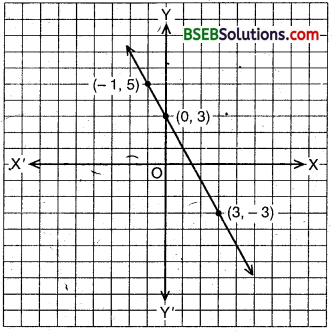Question 2.
Give the equations of two lines passing through (2, 14). How many more such lines are there, aqd why?
Solution:
Here (2, 14) is a solution of a linear equation we are looking for. One example of such a linear equation is 7x – y = 0. Note that x + y = 16, 2x + y = 18 and 7x +y = 28 are also satisfied by the co-ordinates of the point (2, 14). So, any line passing through the point (2, 14) is an example of a linear equation for which (2, 14) is a solution. Thus, there are infinite number of lines through (2, 14).

Question 3.
If the point (3, 4) lies on the graph of the equation 3y = ax + 7, find the value of a?
Solution:
Since (3, 4) lie on the graph corresponding to 3y = ax + 7. Therefore, (3, 4) satisfies the given equation.
That is, 3(4) = a(3) + 7
⇒ 12 – 7 = 3a
⇒ 3a = 5
⇒ a = 53
Hence, for a = 53, (3, 4) lie on the graph of equation 3y = ax + 7.

Question 4.
The taxi fare in a city is as follows : For the first kilometre, the fare is Rs 8 and for the subsequent distance it is Rs 5 per km. Taking the distance covered as x km and total fare as-Rs y, write a linear equation for this information, and draw its graph.
Solution:
Taxi fare for first km = Rs 8
Taxi fare for the subsequent km = Rs 5
Total fare = Rs y
Total distance = x km
The linear equation for the above information is given by y = 8 x 1 + 5(x – 1)
⇒ y = 8 + 5x – 5
⇒ y = 5x + 3
⇒ 5x – y + 3 = 0
When x – 0, y – 5 x 0 + 3 = 0 + 3 = 3
When x = – 1, y = 5x – 1 + 3 = – 5 + 3 = – 2
When x = – 2, y = 5x – 2 + 3 = – 10 + 3 = – 3
Thus, we have the following table :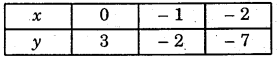Plotting the points (0, 3), (- 1, – 2) and (- 2, – 7) on the graph paper and drawing a line joining them, we obtain the required graph.Question 5.
From the choices given below, choose the equation whose graphs are given in Fig (a) and Fig. (6)
For Figure (a)
(i) y = x
(ii) x + y = 0
(iii) y = 2x
(iv) 2 + 3y = 7x

For Figure (b)
(i) y = x + 2
(ii) y = x- 2
(iii) y = – x + 2
(iv) x + 2y = 6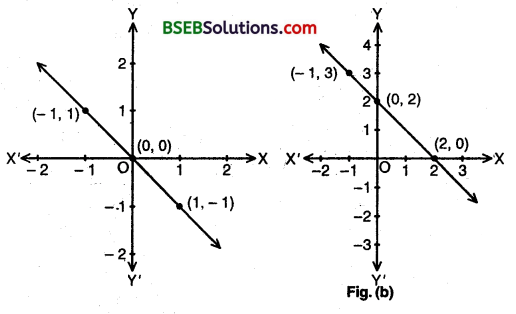Solution:
For Fig. (a), the correct equation from the choices given is clearly x + y = 0 as it is satisfied by the points (- 1, 1) and (1, – 1) given on the graph.
For Fig. (b)y the correct equation from the choices given is clearly y = – x + 2 as it is satisfied by the points (- 1, 3), (0, 2) and (2, 0) given on the graph.

Question 6.
If the work done by a body on application of a constant force is directly proportional to the distance travelled by the body express this in the form of an equation in two variables and draw the graph of the same by taking the constant force as 5 units. Also read from the graph the work done when the distance travelled by the body is (i) 2 units, (ii) 0 unit
Solution:
Let x be the distance and y be the work done. Therefore, according to the problem the equation will bey = 5x.
To draw its graph :
When x = 0, we have, y = 5(0) = 0
When x = 1, we have, y = 5(1) = 5
When x = – 1, we have, y = 5(- 1) = – 5
∴ The table is as under :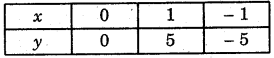Plotting (0, 0), (1, 5) and (- 1, – 5) on the graph paper and drawing a line joining them we obtain the graph of the line y = 5x as shown.
From the graph, clearly,(i) when distance travelled is 2 units,
i.e., x = 2, then y = 10.
∴ Work done = 10
(ii) when distance travelled is 0 units i.e., x = 0, then y = 0.
∴ Work done = 0.

Question 7.
Yamini and Fatima, two students of Class IX of a school, together contributed Rs 100 towards the Prime Minister’s Relief Fund to help the earthquake victims. Write a linear equation which this data satisfies. (You may take their contributions as Rs x and Rs y.) Draw the graph of the same.
Solution:
Let Yamini and Fatima contributed Rs x and Rs y towards the P.M.’s Relief Fund totally Rs 100.
∴ The linear equation using the above data is x + y = 100. i.e., y = 100 – x.
To draw iis graph :
When x = 0, we have y = 100 – 0 = 100
When x = 100, we have y = 100 – 100 = 0
When x = 50, we have y = 100 – 50 = 50
The table for these values is as underPlotting the points (0, 100), (100, 0) and (50, 50) on the graph paper and drawing a line joining them, we obtain the graph of the line x + y = 100 as shown.Question 8.
In countries like the USA and Canada, temperature is measured in Fahrenheit, whereas in countries like India, it is measured in Celsius. Here is a linear equation that converts Fahrenheit to Celsius :
F = (95) C + 32
(i) Draw the graph of the linear equation above using Celsius for x-axis and Fahrenheit for y-axis.
(ii) If the temperature is 30°C, what is the temperature in Fahrenheit?
(iii) If the temperature is 95°F, what is the temperature in Celsius?
(iv) If the temperature is 0°C, what is the temperature in Fahrenheit and if the temperature is 0°F, what is the temperature in Celsius?
(v) Is there a temperature which is numerically the same in both Fahrenheit and Celsius? If yes, find it.
Solution:
(i) We have F = 95C + 32 = ⇒ C = 95(F – 32)
We calculate the values of F for different values of C.
When C = – 40, F = 95 x (- 40) + 32 = – 72 + 32 = – 40
When C= 10, F= 95 x 10 + 32 = 18 + 32 = 50
The table of values is as under :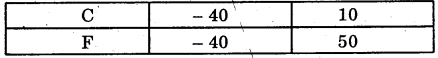We choose a suitable scale on the x-axis (for Celsius) and on the same scale on they-axis (for Fahrenheit).Plotting the points (- 40, – 40) and (10, 50) on the graph point. On joining these points by line segment, we obtain the graph of F = 95C + 32.

(ii) From the graph, we see that when C = 30° shown by C1 on the x-axis in the positive direction, then F = 86 shown by the point F1 on the y-axis in the positive direction.
Hence, 30°C = 86°F.

(iii) From the graph, we see that when F = 95° shown by F2 on the y-axis in the positive direction, then C = 35 shown by the point C2 on the x-axis in the positive direction.
Hence, 95°F = 35°C.

(iv) From the graph, clearly 0°C = 32°F and 0°F = 17.8°F.

(v) Clearly, from the graph, the temperature which is numerically the same in both Fahrenheit and Celsius is – 40° i.e., – 40°C = – 40°F.

## Bihar Board Class 9th Maths Chapter 4 Linear Equations in Two Variables Ex 4.3 Textbooks for Exam Preparations

Bihar Board Class 9th Maths Chapter 4 Linear Equations in Two Variables Ex 4.3 Textbook Solutions can be of great help in your Bihar Board Class 9th Maths Chapter 4 Linear Equations in Two Variables Ex 4.3 exam preparation. The BSEB STD 9th Maths Chapter 4 Linear Equations in Two Variables Ex 4.3 Textbooks study material, used with the English medium textbooks, can help you complete the entire Class 9th Maths Chapter 4 Linear Equations in Two Variables Ex 4.3 Books State Board syllabus with maximum efficiency.

## FAQs Regarding Bihar Board Class 9th Maths Chapter 4 Linear Equations in Two Variables Ex 4.3 Textbook Solutions

#### How to get BSEB Class 9th Maths Chapter 4 Linear Equations in Two Variables Ex 4.3 Textbook Answers??

Students can download the Bihar Board Class 9 Maths Chapter 4 Linear Equations in Two Variables Ex 4.3 Answers PDF from the links provided above.

#### Can we get a Bihar Board Book PDF for all Classes?

Yes you can get Bihar Board Text Book PDF for all classes using the links provided in the above article.

## Important Terms

Bihar Board Class 9th Maths Chapter 4 Linear Equations in Two Variables Ex 4.3, BSEB Class 9th Maths Chapter 4 Linear Equations in Two Variables Ex 4.3 Textbooks, Bihar Board Class 9th Maths Chapter 4 Linear Equations in Two Variables Ex 4.3, Bihar Board Class 9th Maths Chapter 4 Linear Equations in Two Variables Ex 4.3 Textbook solutions, BSEB Class 9th Maths Chapter 4 Linear Equations in Two Variables Ex 4.3 Textbooks Solutions, Bihar Board STD 9th Maths Chapter 4 Linear Equations in Two Variables Ex 4.3, BSEB STD 9th Maths Chapter 4 Linear Equations in Two Variables Ex 4.3 Textbooks, Bihar Board STD 9th Maths Chapter 4 Linear Equations in Two Variables Ex 4.3, Bihar Board STD 9th Maths Chapter 4 Linear Equations in Two Variables Ex 4.3 Textbook solutions, BSEB STD 9th Maths Chapter 4 Linear Equations in Two Variables Ex 4.3 Textbooks Solutions,
Share: